# Python 进行目标检测

02/15 21:27

## 一、前言

从学单片机开始鼓捣C语言，到现在为了学CV鼓捣Python，期间在CSDN、简书、博客园和github这些地方得到了很多帮助，所以也想把自己做的一些小东西分享给大家，希望能帮助到别人。记录人生的第一篇博客，mark。

## 二、图像检测步骤

### 1. 读取两张图片

第一张是需要检测的小物体，第二章图片是小物体放置在大场景中。代码与输出结果如下所示:

``````import numpy as np
import matplotlib.pyplot as plt
import cv2

def my_show(img):
plt.imshow(cv2.cvtColor(img,cv2.COLOR_BGR2RGB))
return

# 读取图片

# 显示图片
plt.figure(figsize=(10,10))
plt.subplot(121)
my_show(img_small)
plt.subplot(122)
my_show(img_big)``````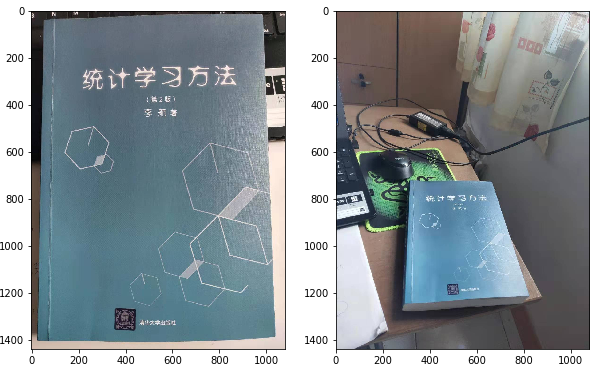### 2. 提取图片中的特征点

这一步就像我们在区分不同的人的时候，一眼看到外貌就知道此人是谁，而外貌就是这个人的特征。我们希望提取该物体的特征点，以便在不同的场景中识别出来。图片是由像素点构成，但是像素点包含的信息太零散了，一般是识别物体的边缘或者角点作为特征信息。常用的特征描述算法如下：

harris corner detection: https://www.jianshu.com/p/efc81fdb8afb

我们项目中用到的是SIFT算法，SIFT精确度高，对尺度、亮度以及旋转的鲁棒性强，不过计算时间长，所需的计算资源较多。SURF是SIFT的加速版本，有兴趣的小伙伴可以了解一下。SIFT算法运行后，可以得到的特征点的位置以及特征向量。（PS：SIFT算法申请了专利，所以在opencv3.4.2版本后不能使用了，需要用SIFT的请安装3.4.2的opencv）

``````import numpy as np
import matplotlib.pyplot as plt
import cv2

def my_show(img):
plt.imshow(cv2.cvtColor(img,cv2.COLOR_BGR2RGB))
return

# 读取图片

# 提取特征点
sift=cv2.xfeatures2d.SIFT_create()
kp1,des1=sift.detectAndCompute(img_small,None)
kp2,des2=sift.detectAndCompute(img_big,None)

# 在图中显示特征点
img_small_sift=cv2.drawKeypoints(img_small,kp1,outImage=np.array([]),flags=cv2.DRAW_MATCHES_FLAGS_DRAW_RICH_KEYPOINTS)
img_big_sift=cv2.drawKeypoints(img_big,kp2,outImage=np.array([]),flags=cv2.DRAW_MATCHES_FLAGS_DRAW_RICH_KEYPOINTS)

plt.figure(figsize=(15,15))
plt.subplot(121)
my_show(img_small_sift)
plt.subplot(122)
my_show(img_big_sift)``````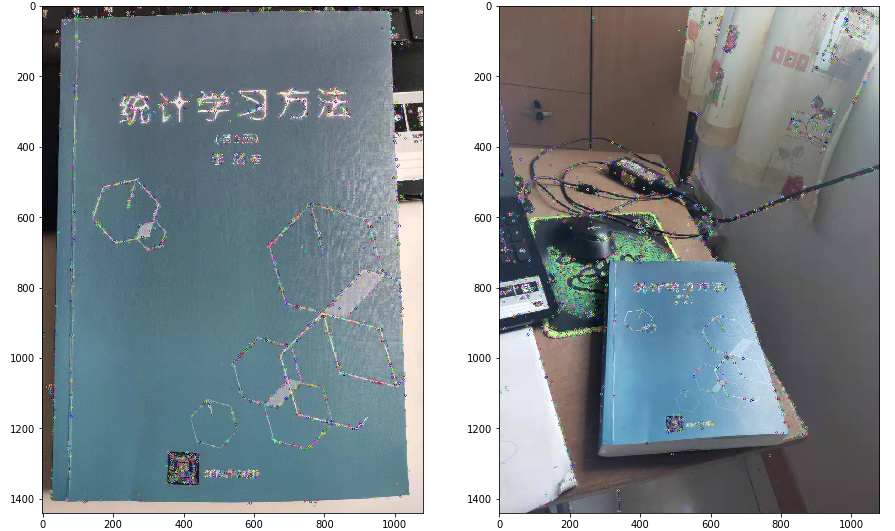### 3.特征点匹配

在分别提取了两张图的特征点后，就需要进行特征点匹配啦。这里需要注意的是，两张图的特征点数量一般情况下是不一样的，opencv算法里默认用第一张图的特征点来匹配,所以匹配矩阵的行数与第一张图特征点的行数一致。常用的匹配方法：

Brute-Force: 暴力搜索，用图1的特征点逐一与图二的特征点比较，找到欧式距离最小的点作为匹配点。不过这样匹配的错误点就会很多，所以常用交叉匹配法消除错误匹配。交叉匹配法指的是，反过来匹配一次，用匹配到图2的点反过来匹配图1的点，如果匹配结果与原来相同，则认为是正确匹配。

KNN: K近邻匹配，在匹配的时候选择K个和特征点最相似的点，如果这K个点之间的区别足够大，则选择最相似的那个点作为匹配点，通常选择K = 2。KNN匹配也会出现一些误匹配，这时候需要对比第一邻近与第二邻近之间的距离大小，假如 distance_1< (0.5~0.7)*distance_2, 则认为是正确匹配。

FLANN: FLANN是快速最近邻搜索包（Fast Library for Approximate Nearest Neighbors）的简称，是最近邻搜索的算法的集合。

一般我们会选择调用cv2.Brute-Force或者cv2.FlannBasedMatcher来进行特征点匹配，FLANN里边就包含的KNN、KD树还有其他的最近邻算法。

### 4.计算单应性矩阵

这里我们需要在大场景中用矩形框出匹配的小物体，所以就要计算单应性矩阵，然后做投影变换。RANSAC（Random Sample Consensus随机抽样一致性算法是计算单应性矩阵的有效方法，并且在寻找单应性矩阵的过程中可以进一步剔除错误匹配点。RANSAC算法的步骤如下：

•  随机抽取四个匹配点对计算投影矩阵，需要检验四个点是否共线
•  图片1特征点坐标齐次变换后（3，1）乘上投影矩阵（3，3），然后计算变换后的特征点坐标与图片2特征点坐标的欧氏距离，小于设定的阈值则记录为内点，反之则为内点
•  判断此次内点的数量是否比以往记录的内点最大值多，如果是，则更新投影矩阵，如果不是，则不更新
•  判定循环次数是够达到设定次数或内点数占全部匹配点的比例达到设定比例，则跳出循环，否则跳到步骤1继续循环

寻找到最优的单应性矩阵后，将框住物体一的矩阵经投影变换后在图片二上画出来，完成目标检测框选。以下代码摘自Brook@CV的博客并修改，如有侵权，请通知删除

``````import numpy as np
import cv2
from matplotlib import pyplot as plt

MIN_MATCH_COUNT = 10

# 使用SIFT检测角点
sift = cv2.xfeatures2d.SIFT_create()
# 获取关键点和描述符
kp1, des1 = sift.detectAndCompute(img1,None)
kp2, des2 = sift.detectAndCompute(img2,None)

# 定义FLANN匹配器
index_params = dict(algorithm = 1, trees = 5)
search_params = dict(checks = 50)
flann = cv2.FlannBasedMatcher(index_params, search_params)
# 使用KNN算法匹配
matches = flann.knnMatch(des1,des2,k=2)

# 去除错误匹配
good = []
for m,n in matches:
if m.distance <= 0.7*n.distance:
good.append(m)

# 单应性
if len(good)>MIN_MATCH_COUNT:
# 改变数组的表现形式，不改变数据内容，数据内容是每个关键点的坐标位置
src_pts = np.float32([ kp1[m.queryIdx].pt for m in good ]).reshape(-1,1,2)
dst_pts = np.float32([ kp2[m.trainIdx].pt for m in good ]).reshape(-1,1,2)
# findHomography 函数是计算变换矩阵
# 参数cv2.RANSAC是使用RANSAC算法寻找一个最佳单应性矩阵H，即返回值M
M, mask = cv2.findHomography(src_pts, dst_pts, cv2.RANSAC,5.0)
# ravel方法将数据降维处理，最后并转换成列表格式
# 获取img1的图像尺寸
h,w,dim = img1.shape
# pts是图像img1的四个顶点
pts = np.float32([[0,0],[0,h-1],[w-1,h-1],[w-1,0]]).reshape(-1,1,2)
# 计算变换后的四个顶点坐标位置
dst = cv2.perspectiveTransform(pts,M)

# 根据四个顶点坐标位置在img2图像画出变换后的边框
img2 = cv2.polylines(img2,[np.int32(dst)],True,(0,0,255),3, cv2.LINE_AA)

else:
print("Not enough matches are found - %d/%d") % (len(good),MIN_MATCH_COUNT)

# 显示匹配结果
draw_params = dict(matchColor = (0,255,0), # draw matches in green color
singlePointColor = None,
flags = 2)
img3 = cv2.drawMatches(img1,kp1,img2,kp2,good,None,**draw_params)
plt.figure(figsize=(20,20))
plt.imshow(cv2.cvtColor(img3,cv2.COLOR_BGR2RGB))
plt.show()``````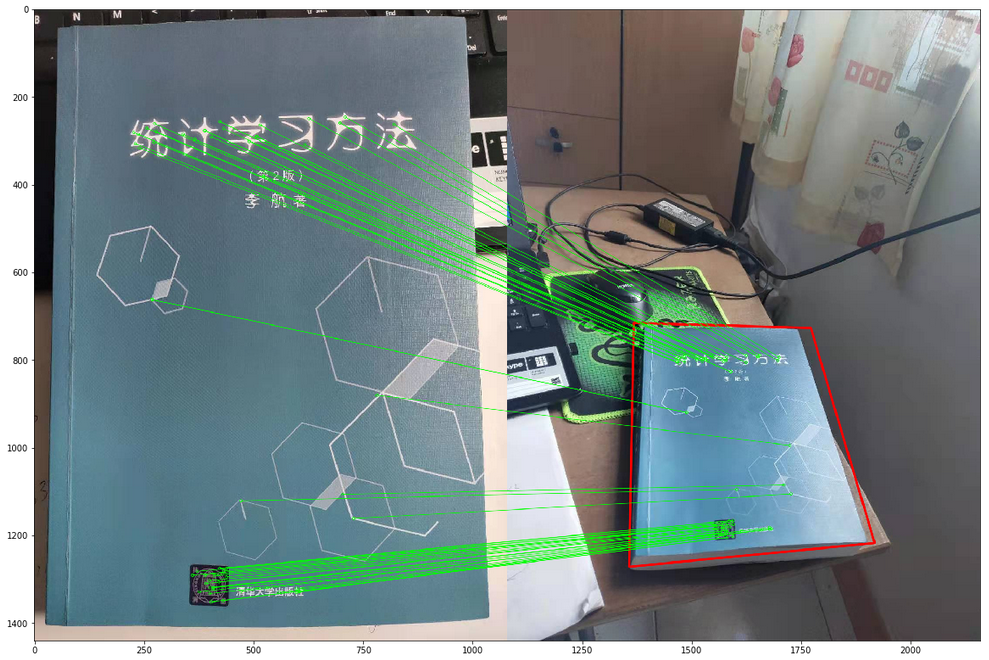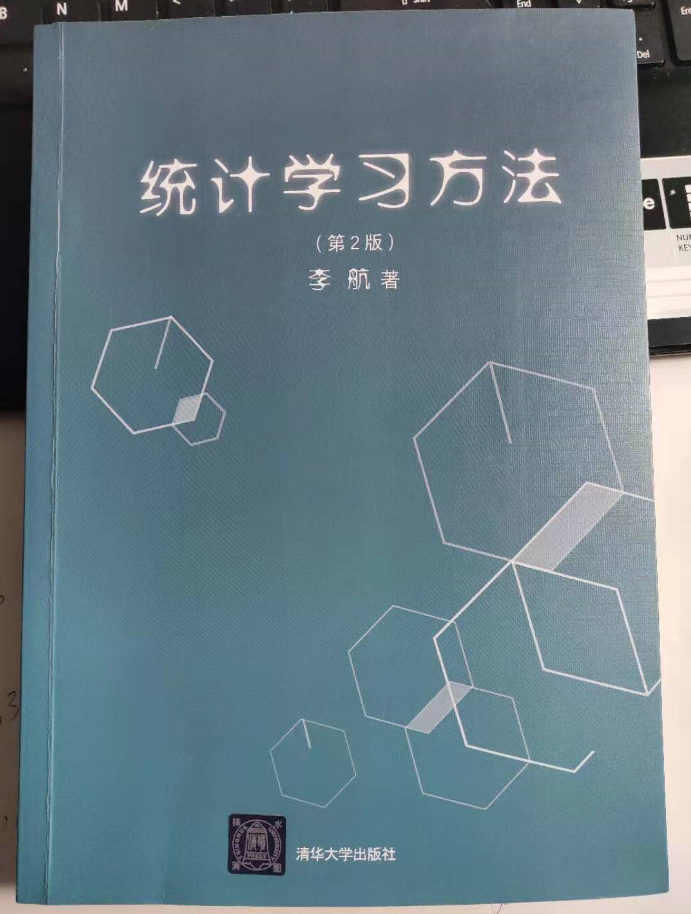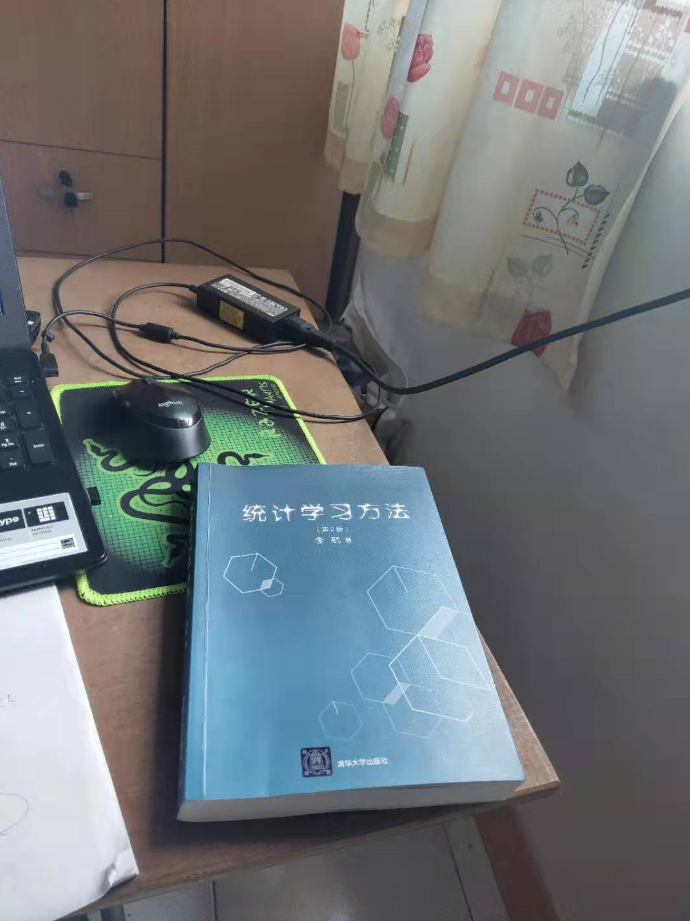0
0 收藏

### 作者的其它热门文章0 评论
0 收藏
0